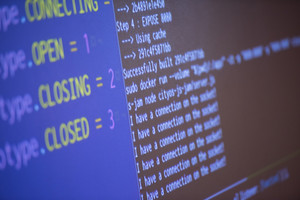# Binary To Decimal Converter

Enter the binary number to decode, and then click "Convert!":

The decoded number:

Please note: any spaces or colons (:) in the binary string will be removed.

Need to convert decimal to binary?

## How Do You Use The Binary To Decimal Online Tool?

Converting binary to decimal online is quite easy. First, you will need to enter the binary number you would like to decode in the first text box, then click the 'convert!' button. The Binary decoder tool will then display the converted number into the decimal form. There is no limit to the number of times you can use the converter tool so feel free to use it as much as you need.## What is the Binary System?

In the binary system, numbers are made up of only 0s and 1s. For example, a binary number may be 10101, but when converted to decimal the value is 21. This is because the binary system uses the two symbols, 0 and 1, to represent the numeric values that we are commonly used to. This system dates back to the 17th century but is still used today in all modern computers. Programmers utilize the binary system by using the two symbols to represent numbers that are capable of being in two mutually exclusive states, on or off. There are two common methods for converting binary to decimal - Positional Notation & Doubling. The easiest & most efficient method is of course, using the String-Functions binary to decimal converter tool. Use our online Decimal to Binary Converter Tool here!Keywords: binary decode a string text, tool, on line tool, tech career decoder.
String Manipulation For Programmers
For a comparison of string function notation in different programming languages such as Pascal, VB.NET, Perl, Java, C, C++, Ruby and many more, see the Wikipedia article Comparison Of Programming Languages (String Functions)

Did We Miss a Conversion Tool? Let Us Know!